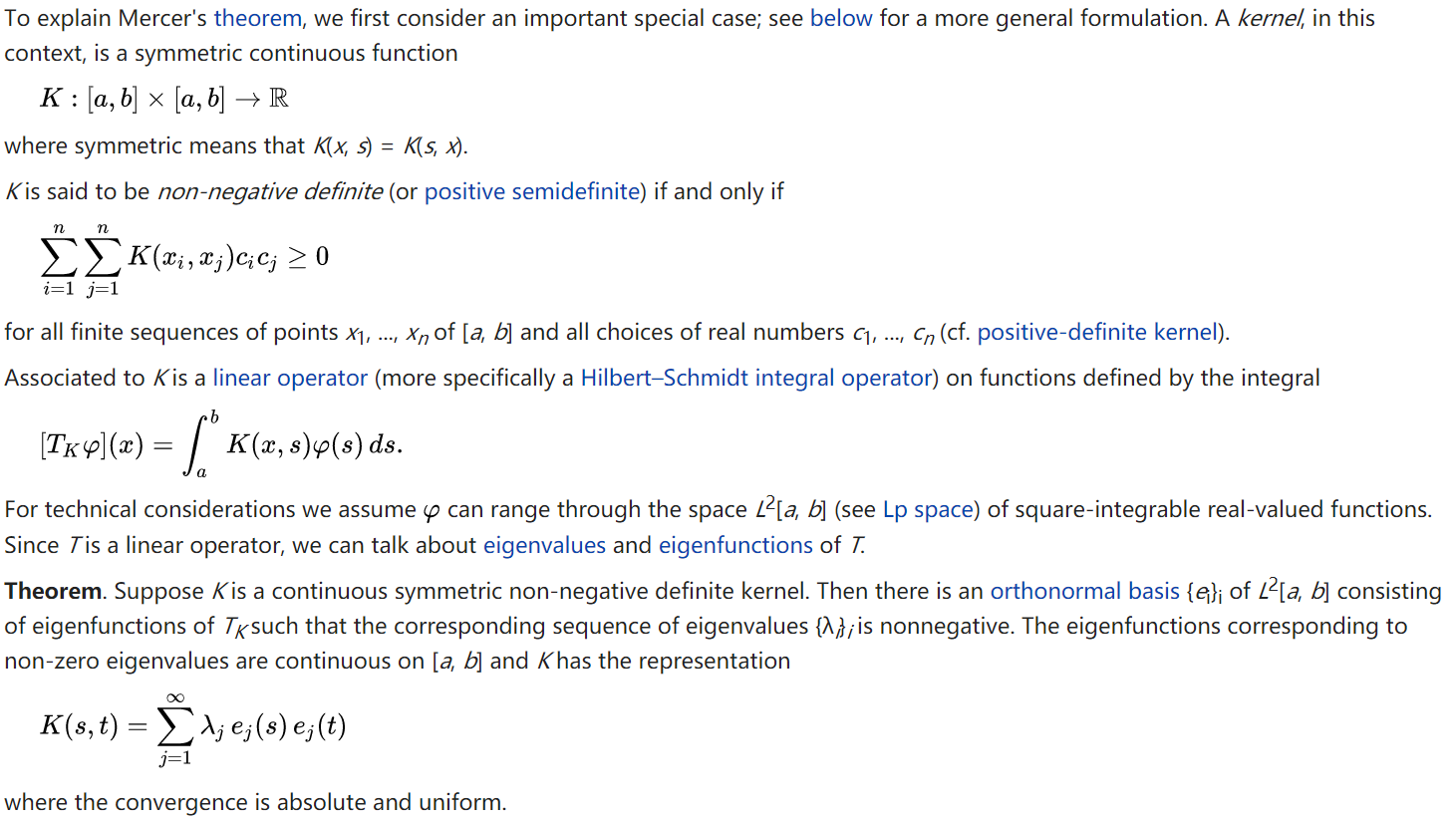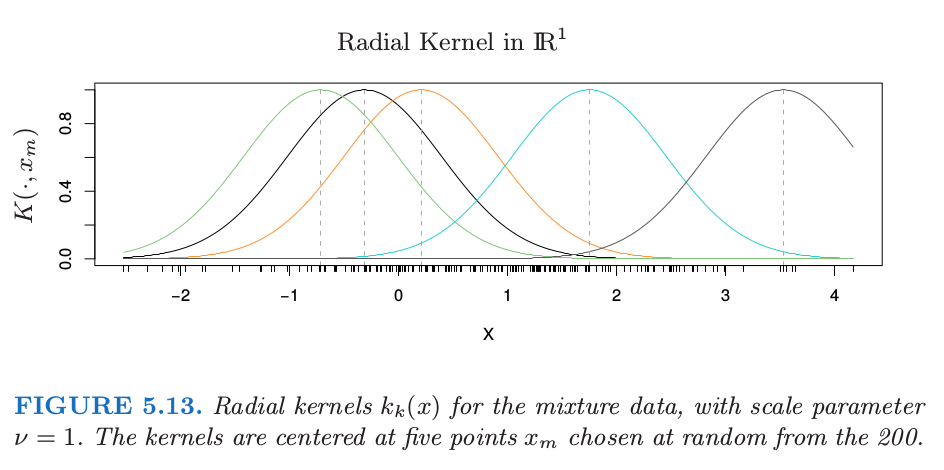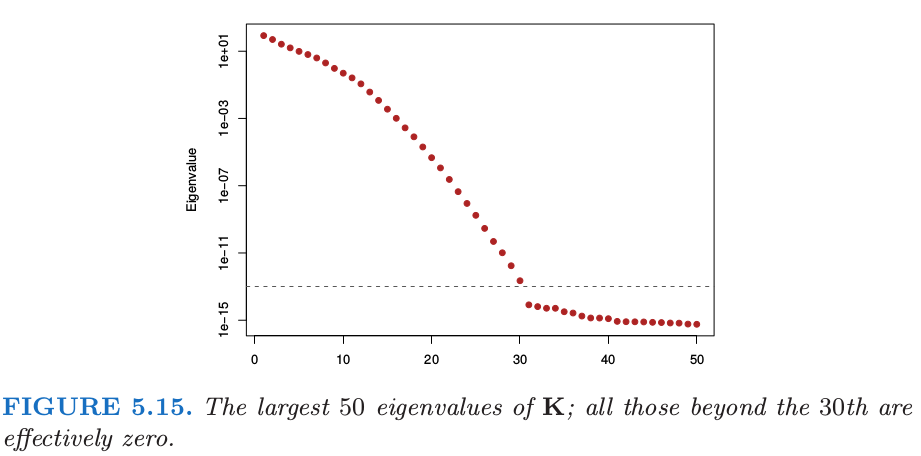5.8 正则化和再生核希尔伯特空间理论¶

核产生的函数空间¶

weiya 注：Mercer’s theorem

\eqref{5.45} 的分解由 Mercer’s theorem 保证，Mercer’s theorem 将半正定矩阵的特征分解推广到半正定核函数的特征分解，具体地，图片来源: Wiki: Mercer’s theorem

${\cal H}_K$ 的元素是关于这些 特征函数 (eigen-functions) 的展开，即

weiya 注：Induced Norm

weiya 注：两种构造 RKHS 的方法

\eqref{5.42} 中空间 ${\cal H}_K$ 的惩罚函数定义为二次范数 $J(f)=\Vert f\Vert_{{\cal H}_K}^2$．$J(f)$ 的值可以解释为广义岭惩罚，其中在展开式 \eqref{5.45} 中，大的特征值惩罚较小，反之亦然．

weiya 注：Ex. 5.15

weiya 注: 核再生性质 (kernel reproducing property)

RKHS 的例子¶

$N$ 个拟合值的向量为

weiya 注：Recall

带惩罚的多项式回归¶

weiya 注：

weiya 注：Ex. 5.16

This simplicity is not without implications. \eqref{5.61} 中的每个多项式 $h_m$ 从 $K$ 的特定形式继承了缩放因子，这对 \eqref{5.63} 的惩罚有影响．我们将在下一节详细讨论．

高斯径向基函数¶$\Phi$ 的第 $\ell$ 列是 $\phi_\ell$ 的一个估计，这是对 $N$ 个观测中的每一个进行赋值．另外，$\Phi$ 的第 $i$ 行是基函数 $\phi(x_i)$（在 $x_i$ 处取值）的估计向量．尽管原则上，$\phi$ 可以有无穷多的元素，但我们的估计至多有 $N$ 个元素．weiya 注：Ex. 5.17

支持向量机¶

1. Girosi, F., Jones, M. and Poggio, T. (1995). Regularization theory and neural network architectures, Neural Computation 7: 219–269.

2. Wahba, G. (1990). Spline Models for Observational Data, SIAM, Philadelphia.

3. Evgeniou, T., Pontil, M. and Poggio, T. (2000). Regularization networks and support vector machines, Advances in Computational Mathematics 13(1): 1–50.

4. Cressie, N. (1993). Statistics for Spatial Data (Revised Edition), Wiley-Interscience, New York.

5. Vapnik, V. (1996). The Nature of Statistical Learning Theory, Springer, New York.

6. Hastie, T. and Zhu, J. (2006). Discussion of “Support vector machines with applications” by Javier Moguerza and Alberto Munoz, Statistical Science 21(3): 352–357.